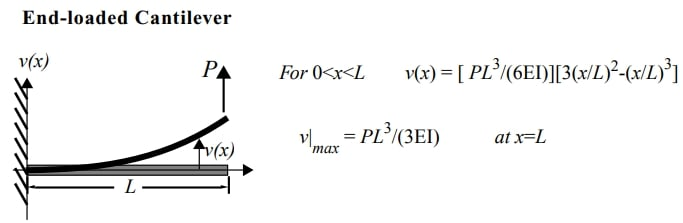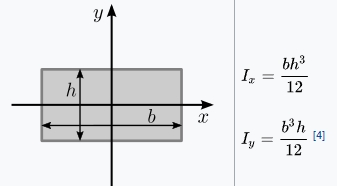## 3D Design

•chukster97
Subscriber

hello im very new to ansys mechanical apdl, im trying to create a simple model for my project "VM5 is the code for ansys model", a beam with a load on the end. can someone create a simple model with log file to show me how it works.

•peteroznewman
Subscriber

For example what is the beam

• length

• cross-section

• material

Do you have to use APDL or can you use Workbench?

•chukster97
Subscriber

the length can be 30mm with a 5mm cross section, the material can be shell181 with a load of 10N. i wish to use ADPL because its the software i learnt to use in university, thank you.

•peteroznewman
Subscriber

What is the shape of the cross-section?  A circle, a square. You have only given a dimension.

shell 181 is not a material. A material is steel and has an Elastic Modulus.  What is the Elastic Modulus?

•chukster97
Subscriber

the young modulus is 200gpa, is it possible to port workbench models to adpl?

•peteroznewman
Subscriber

Yes, but if you start in Workbench, you can complete the project in Workbench.

•chukster97
Subscriberthis is the link to the model im trying to create just the basic drawing, theres two of them with a cylinder as a second and a load of 10N.

•peteroznewman
Subscriber
•peteroznewman
Subscriber

Do you have the theoretical solution to a 10 N load on the 10 mm square cross-section 50 mm long beam?

Get started by creating that beam solid in SpaceClaim.

•chukster97
Subscriber

i have no theoretical solutions, i just need help to make this. is it possible to model it then send a download link so i can run the simulation calculations i need? thanks

•peteroznewman
Subscriber

Here is the equation to calculate the tip displacement with an end load for a uniform section cantilever beam provided by a lecture at MIT.Do you know how to calculate I for the square cross-section?  Wikipedia has that equation.In your case, h = b and Ix = Iy.

Next start a beam model in ANSYS to see if you get the same answer.

You will need to open SpaceClaim. Draw a line 50 mm long, and create a beam with a square profile with a 10 mm side length.

•chukster97
Subscriber

i told you i have no idea how to use it, doesnt matter i'll ask someone else.

•peteroznewman
Subscriber

I'm here to help you learn how to use ANSYS.  I assume you have installed the software.

• Step 1. Open Workbench.

• Step 2. Double click on the Static Structural analysis system in the Toolbox.

• Step 3. Double click on the Geometry cell in the Static Structural system.

• Step 4. Draw a line 50 mm long in SpaceClaim.

If you can accomplish these few steps, we can cover the next few steps.